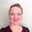# Scaffolding Strategies: Examples in a 4th Grade Geometry Unit @ Caldwell Elementary School, by Sa...Sara Glascock
Get Started. It's FreeScaffolding Strategies: Examples in a 4th Grade Geometry Unit @ Caldwell Elementary School, by Sara Glascock## 2. Related Objectives to the Standard:

### 2.1. -Students will learn the following unit vocabulary: angle, degrees, endpoint, line segments, right angle, acute angle, obtuse angle, ray, lines, protractor, parallel lines, perpendicular lines, and right triangle.

2.1.1. Scaffolding Strategy: Cue Cards:Prepared cards given to individual or groups of students to assist in their discussion about a particular topic or content area: Vocabulary words to prepare for exams; content-specific stem sentences to complete; formulae to associate with a problem; concepts to define. *In this case students could create their own cards.

### 2.2. -Students will obtain the following enduring understandings: geometry and spatial sense offer ways to interpret and reflect on our physical environment, analyzing geometric relationships develops reasoning and justification skills, transforming an object does not affect its attributes, and that points, lines and planes are the foundation to geometry.

2.2.1. Scaffolding Strategy: Think-Pair-Share: All learners need time to process new ideas and information. They also need time to verbally make sense of and articulate their learning with the community of learners who are also engaged in the same experience and journey. Students could also use a graphic organizer as a part of this activity.

### 2.3. -Students will gain the ability to use a protractor, including: measuring angles in whole-number degrees and sketching angles of specified measure.

2.3.1. Scaffolding Strategy: Handouts: Prepared handouts that contain task- and content-related information, but with less detail and room for student note taking. *In this case the handout could have explicit directions for how to use a protractor.

### 2.4. -Lines of symmetry: Students will be able to state a figure is symmetric when it can be divided by at least one line into two congruent parts where the two parts are mirror images of one another…demonstrate that a line of symmetry is a line on which a figure can be folded so the two parts match exactly…explore how a figure can have more than one line of symmetry…and identify shapes that are symmetric.

2.4.1. Scaffolding Strategy: Fish Bowl Activity: A small group of students in the center are circled by the class as the group in the middle, or fishbowl, engaging in an activity, modeling how it's done for the larger group. *In this case a myriad of activities could be emplyed to demonstrate how to identify llines of symmetry.

### 2.5. -Students will recognize angle measure as additive, meaning, when an angle is decomposed into non-overlapping parts, the angle measure of the whole is the sum of the angle measures of the parts…Students will be able to solve addition and subtraction problems to find unknown angle measurements on a diagram…Students will be able to write an equation with a symbol for an unknown angle measure.

2.5.1. Scaffolding Strategy: Show Examples: Samples, specimens, illustrations, problems: Real objects; illustrative problems used to represent something. *In this case the teacher could use the hands of a clock as an example of demonstrating angles.

### 2.6. -Students will be able to classify two-dimensional figures based on the presence or absence of parallel or perpendicular lines, or the presence or absence of angles of a specified size.

2.6.1. Scaffolding Strategy: Examples: Samples, specimens, illustrations, problems: Real objects; illustrative problems used to represent something. *Students will be given examples and will be required to provide examples.

## 4. Key Factors about Caldwell Students to Inform Strategy Selection

### 4.2. Prior knowledge about the topic students will need to build on:

4.2.1. - Students will understand that shapes in different categories (e.g., rhombuses, rectangles, and others) may share attributes (e.g., having four sides), and that the shared attributes can define a larger category (e.g., quadrilaterals).

4.2.2. -Students must know and be able to identify the following (taught in previous grades): Cube Half/Quarter Circle, Hexagon, Pentagon, Polygon, Quadrilateral, Rectangle Rhombus/Rhombi, Square, Trapezoid,Triangle

4.2.3. -Students will recognize rhombuses, rectangles, and squares as examples of quadrilaterals, and draw examples of quadrilaterals that do not belong to any of these subcategories.